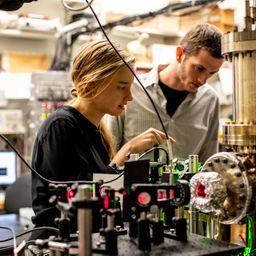## a. 2.21 \times 10^{-32} \mathrm{kg.m} / \mathrm{s}b. 1.00 \times 10^{10} \mathrm{Hz}c. 4.14 \times 10^{-5} \mathrm{eV}

Quantum Physics

Atomic Physics

### Discussion

You must be signed in to discuss.
##### Top Physics 103 Educators##### Christina K.

Rutgers, The State University of New Jersey##### Andy C.

University of Michigan - Ann Arbor### Video Transcript

it's so for this problem. We know that we have a microbe proton and its wavelength is three centimeters. We'll go ahead and record that information and we want the mo mentum frequency and energy of the proton. So the energy of a Eric Sorry. Let's start with actually the momentum. So he is, well, Thio. He is equal to H over Lambda, which was given in the book. And so we just want to plug in this for Lambda and and for H. Of course it's Planck's constant. And then for that we want to plug in, um 6.626 times have minus 34 culturally proud right now, so dividing consumption by 340.23 And for that I got to point c Hominy sig figs 2.21 times 10 to the minus 32. And then these air both ass I So I can use the s i unit for momentum in S i u nit, I guess kilogram meters per second. And for be what? We want the frequency. So, um, you want to use f is equal to see divided by Lambda. And for that, I'll always remember that by unit analysis 2.99 meat times 10 to the it right with Franco. Three stretch, um, 9.99 times 10 of the nine. All right, Okay, hurts. And first, see, what we want to get is the energy. So it is equal to H f. So we just want to evaluate, um, this frequency multiplied by Planck's constant. So I'll go ahead and do that in another tab. And I got I got 6.62 times, 10 to the minus 24 jewels. And then do I need to convert that? Oh, yeah. I mean, electron volts. So, um, then we need to divide by the conversion. One point of 1.602 Turn the minus 19. So that's 4.13 e. B times having minus. Hi, Bebe. Double check that numberUniversity of Washington

#### Topics

Quantum Physics

Atomic Physics

##### Top Physics 103 Educators##### Christina K.

Rutgers, The State University of New Jersey##### Andy C.

University of Michigan - Ann Arbor